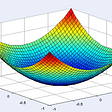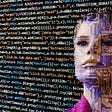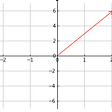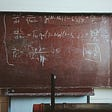# A Neural Net From Scratch

This post is an example of regression, where we will use supervised learning (a model that returns a function after giving it an input and output). The goal is to find the temperature conversion equation. The complete code is at the end of the article.

First, we import NumPy, then defining the training set x_train and y_train. x_train is the independent variable representing temperature in Celsius, while y_train is the dependent variable representing the temperature in Fahrenheit.

x_train is divided by zero because large numbers are not stable; try to run the model without it for 100 epochs, and the loss function will return inf.

`import numpy as npx_train = np.array([[-40.], [-30], [-20], [-10], , , , , ])/100y_train = np.array([31.28, 31.46, 31.64, 31.82, 32., 32.18, 32.36, 32.54, 32.72])`

The code has five functions: forward, backward, loss, step, and model.

Function 1: forward()

The function returns the multiplication of the parameter weight with the dependent variable and adding the parameter bias.

`def forward(w, x, b): return np.float(w*x+b)`

Function 2: backward()

This function calculates the gradients; it guides the model in the right direction.

`def backward(x, y, pred, dw, db):    dw = dw + (-2)*x*(y-pred)    db = db + (-2)*(y-pred)    return dw, db`

Know how did we come up with this equation?

It’s the partial derivative of the loss function, more on calculus in this article:

Function 3: loss()

This example uses Mean Squared Error (MSE) as the loss function.

`def loss(y, pred): return np.array(np.square(y-pred).mean())`

Function 4: step()

The optimizer step uses the learning rate and gradients to update the parameters.

`def step(w, b, dw, db, lr=.01):    w = w - lr * dw    b = b - lr * db    return w, b`

Function 5: model()

The model calls all the functions above since we have a single neuron with two parameters, a weight, and a bias.
At the nested loop, we zero the gradients because every epoch is a fresh start. Then we call the forward and the backward function, followed by printing the loss and updating the parameters. Code completed!

`def model(x, y, epoch=10):    m = x.shape    w = 1.    b = 0    for i in range(epoch):        print(f'----------epoch: {i}-------')        dw, db = 0, 0        for j in range(m):            pred = forward(w, x[j], b)            dw, db = backward(x[j], y[j], pred,dw, db)        print(f'loss: {loss(y, pred)}')        w, b = step(w,b,dw,db, lr=.1)    return w, b`

After running the code for 50 epochs, the loss function got minimized.

`>>> w, b = model(x_train, y_train, 50)----------epoch: 0-------loss: 998.7760000000001----------epoch: 1-------loss: 678.2142745600001----------epoch: 2-------loss: 400.5283809648645...----------epoch: 47-------loss: 0.7345516170916814----------epoch: 48-------loss: 0.7323773915041649----------epoch: 49-------loss: 0.7343447652825804`

The result, w =~1.8 and b =~32, the constants we were after.

`>>> print(w, b)1.7986596334086586 31.999543280738333`

Now to convert from Celsius to Fahrenheit, you can reuse the forward function as a calculator, for example:

`>>> forward(w, 100, b)211.8655066216042`

Now, is the correct way to solve a linear function, no. But, It is a simple exercise for beginners. I hope that helps.

--

--

--

## More from Mansoor Aldosari

https://github.com/booletic

Love podcasts or audiobooks? Learn on the go with our new app.

## Understanding Deep Learning through Energy Landscapes## Natural Langage Processing- Business use cases## Beginner Guide to Machine Learning Pipeline Monitoring## Climbing Mount Rainier- From a Machine Learning Point of View## Copista: Training models for TensorFlow Mobile## Convolutional Neural Networks for Medical Image classification## Recognizing Contextual Entailment using Nneural Network in NLP## How to learn Machine Learning using the BCTI method in 2022 | Allin1hub## Mansoor Aldosari

https://github.com/booletic

## Model Visualization## Linear Algebra with Numpy- Part 1## Explicated Linear Algebra using NM Dev Libraries## Simple Conjectures, Difficult Proofs